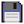# Bit (API)

The Bit API is for manipulating numbers using bitwise binary operations.

All of these functions treat the numbers as 32-bit integers, and will raise an error if an input number is greater than 4,294,967,295.Bit (API)
Function Return values Description
bit.blshift(number n, number bits) number value Shifts a number left by a specified number of bits.
bit.brshift(number n, number bits) number value Shifts a number right arithmetically by a specified number of bits.
bit.blogic_rshift(number n, number bits) number value Shifts a number right logically by a specified number of bits.
bit.bxor(number m, number n) number value Computes the bitwise exclusive OR of two numbers.
bit.bor(number m, number n) number value Computes the bitwise inclusive OR of two numbers.
bit.band(number m, number n) number value Computes the bitwise AND of two numbers.
bit.bnot(number n) number value Computes the bitwise NOT of a number.

# Deprecated Functions

 These functions have been deprecated.These functions have been removed from ComputerCraft .Bit (API)
Function Return values Description
bit.tobits(number n) table bit_tbl Converts a number to an array (numerically-indexed table) containing the corresponding binary bit values.
bit.tonumb(table bit_tbl) number value Converts an array (numerically-indexed table) of 0 and 1 values representing a number in binary into that number.